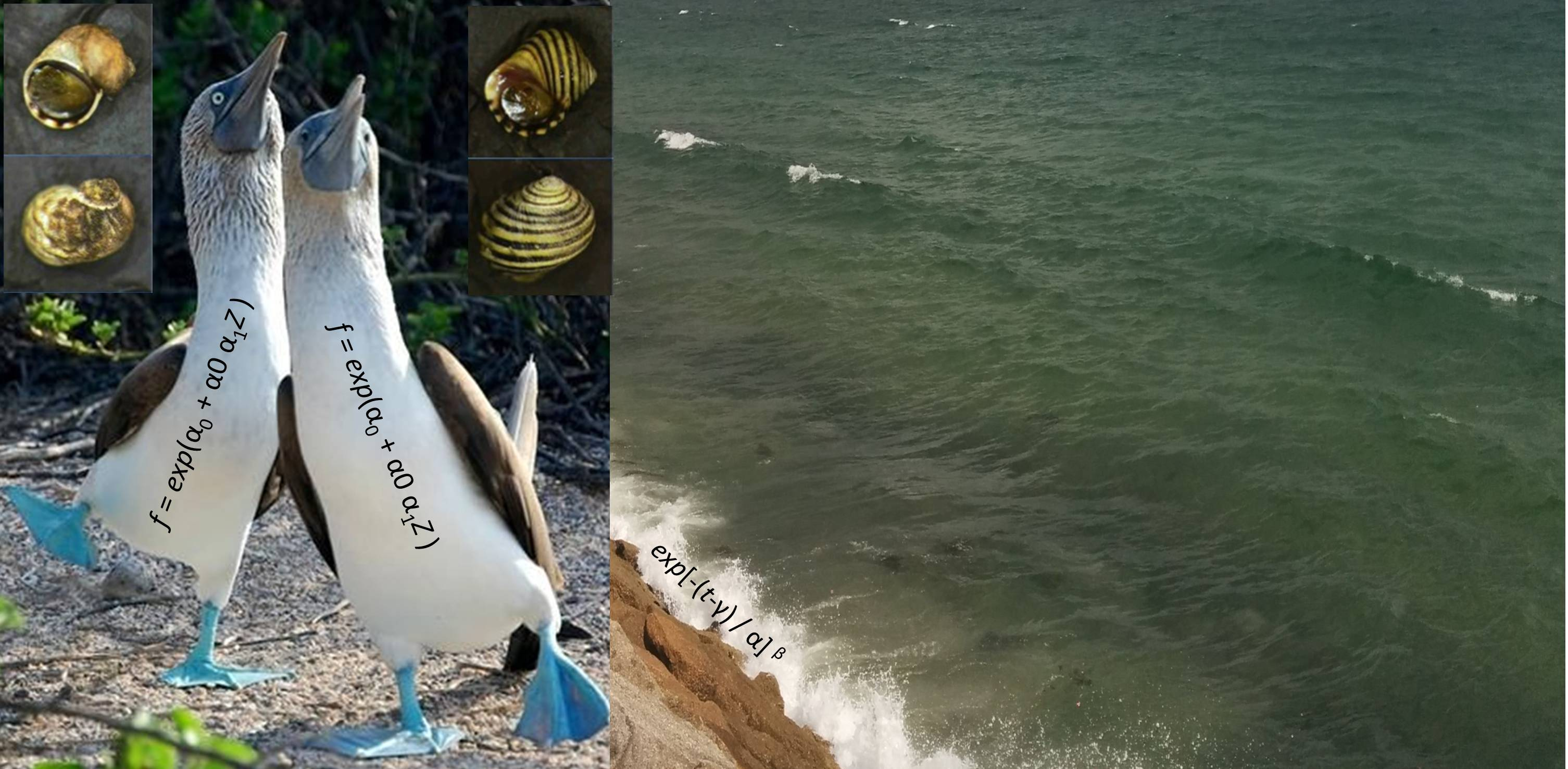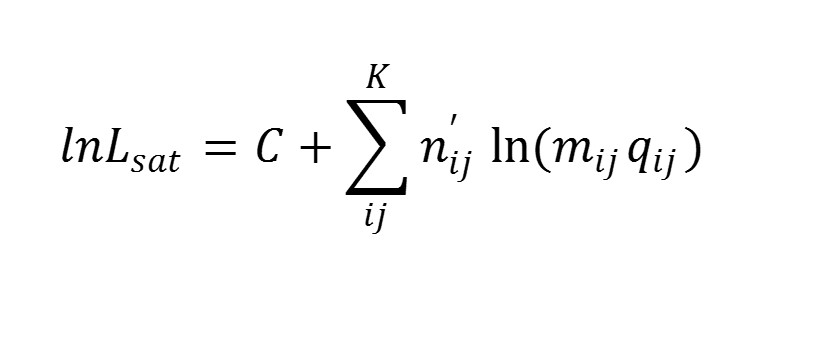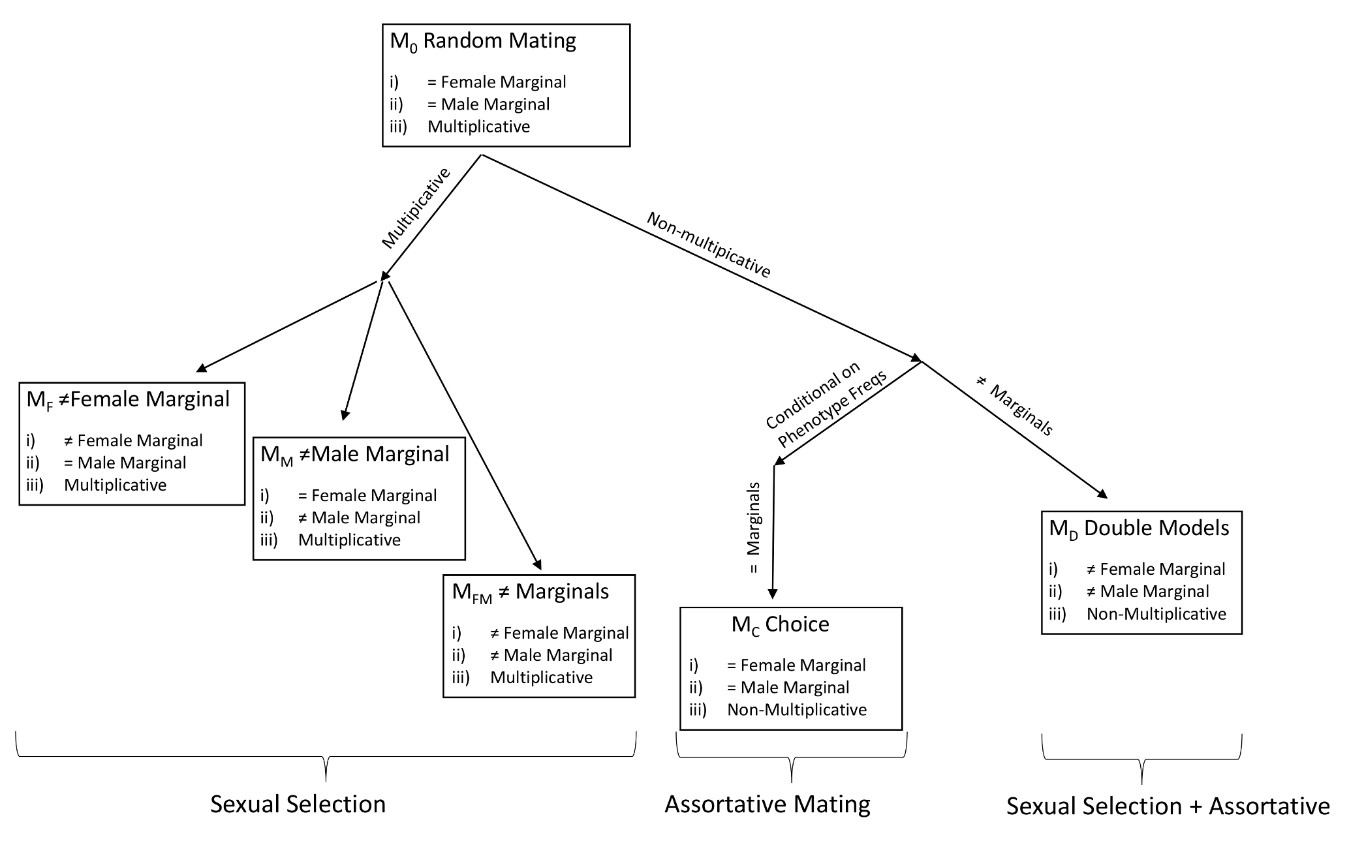QInfoMating: Sexual selection and assortative mating estimation
Mate choice effects models
Given an encounter between an i-type female and a j-type male, let the probability of mating to depend on the mutual mating propensity mij. Therefore, the mating probability between types i and j is expressed as q'ij = mijqij where qij is the encounter probability i.e. the product of the population female and male frequencies of each type (qij = p1ixp2j = (n1in2j) / (n1n2) and n1i is the number of females of type i from the total of n1 females in the population and n2j is the number of males j from n2 males in the population). The values mij are normalized so that the summatory q'ij = 1 (Carvajal-Rodriguez 2018).

Therefore, consider a sample of n' matings between different types of females and males. If there are k1 types of females and k2 types of males then the total number of possible matings is K = k1 x k2. Let have a sample with n'ij matings of i type female with j type male. If the probability of the mating i x j is q'ij, the logarithm of the likelihood function of the sample iswhere C is the multinomial coefficient which is constant given the mating data. The maximum likelihood estimator (MLE) of the probability of the mating i × j is n'ij / n' and if we consider the population frequencies qij as already known, then the MLE of mij corresponds to the Pair Total Index PTI(i,j).

We say this model Msat is saturated because it has as many parameters as data categories in the sample i.e. it is the most complex model that can be fitted to the available data. Interestingly, it is possible to show that the random mating model M0 corresponds to Msat subjected to the following restrictions:

1. Multiplicative effects: mij= mFem_ii × mMale_j for every i,j
2. Equal female marginals: mFem_i = mFem_j for every i,j
3. Equal male marginals: mMale_i = mMale_j for every i,j

It is useful to express the random mating model in such a way because we can generate particular models of interest by relaxing some of the conditions.Thus, we can develop a set of hypotheses corresponding to different mate choice and intrasexual competition effects and then using data-based evidence for ranking each hypothesis and perform multi-model-based inference. The QInfoMating program performs this task.

A. Carvajal-Rodriguez - Departamento de Bioquímica Genética e Inmunología - Universidad de Vigo. ( Last update: August 2023)